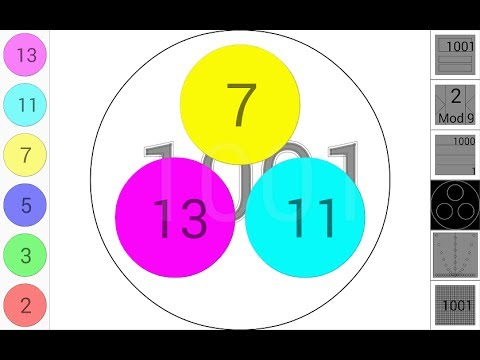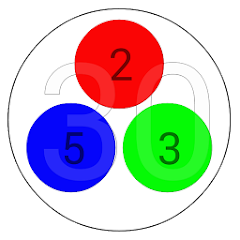# Touch Natural Numbers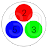1K+Everyone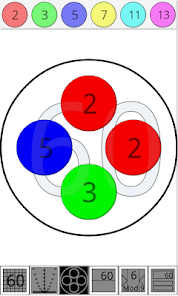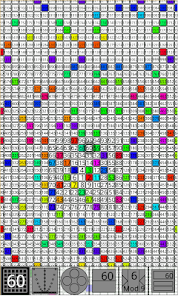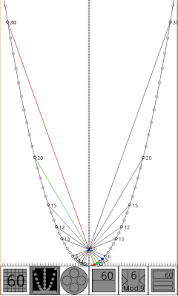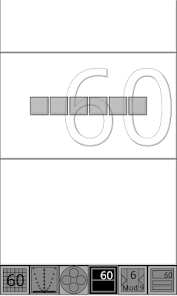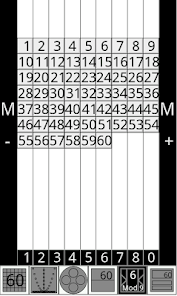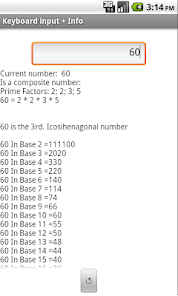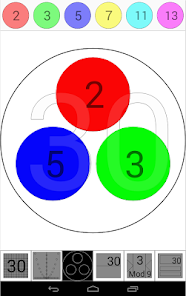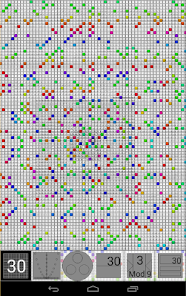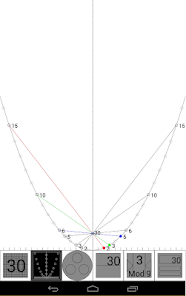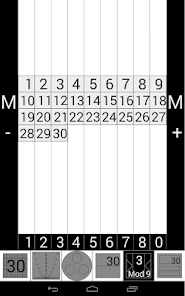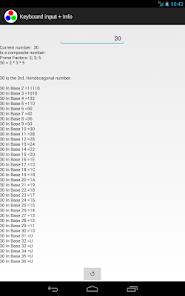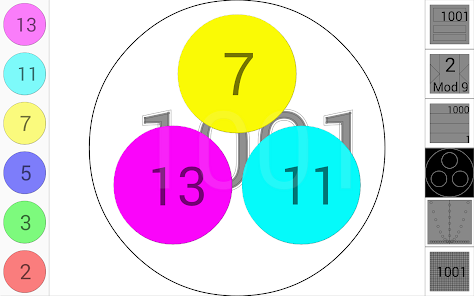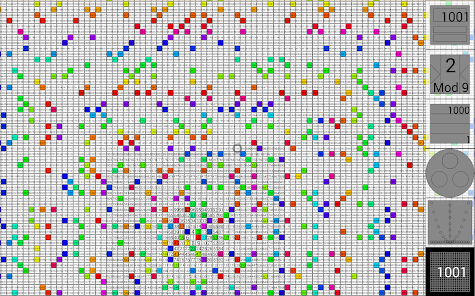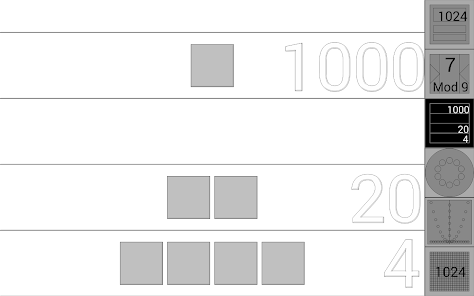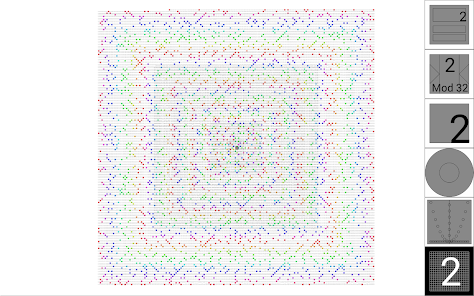Small laboratory of Natural Numbers:
The Prime numbers are the building blocks of the numbers.
With this app you can:
Analyze the factors of a Composite number.
( Prime factorization of all the composite numbers between 1 and 28181: Factoring natural numbers: Prime factors).
Build a Composite number from its factors. (multiplication and division of Natural Numbers)
Localize or select a number in the Ulam Spiral.
(Only the Prime Numbers have a color in this spiral).
Verify all the possible products between Primes in a composite number with the Parabolic Sieve (Yuri Matiyasevich & Boris Stechkin).
Analyze or build a number with the Place Value activity (Addition and Subtraction of Natural Numbers)
Understand the Modulo of a number.
Know about the polygonal numbers and the numbers as the sum of two prime numbers, and as the sum of three Prime Numbers.

http://nummolt.blogspot.com.es/2014/06/touch-natural-numbers.html

And:
The fundamental theorem of arithmetic, also called the unique factorization theorem or the unique-prime-factorization theorem, states that every integer greater than 1 either is prime itself or is the product of prime numbers, and that this product is unique, up to the order of the factors

And Goldbach Conjecture. (Every even integer greater than 2 can be expressed as the sum of two primes)

Touch Math Apps:
http://www.nummolt.com
Nummolt apps: "Not Montessori per se, but Montessori-like"

Registered at MathTools ( MathForum )
http://mathforum.org/mathtools/tool/240793/
Courses:
* Math 1 - Place value
* Math 2 - Place value
* Math 3 - Addition, Subtraction, Multiplication, Division, Whole numbers, Place value, Prime, Composite, Factor, Prime factorization, Divisibility tests, Multiple
* Math 4 - Addition, Subtraction, Multiplication, Division, Whole numbers, Place value, Prime, Composite, Factor, Prime factorization, Divisibility tests, Multiple
* Math 5 - Addition, Subtraction, Multiplication, Division, Whole numbers, Properties of numbers, Prime, Composite, Factor, Prime factorization, Divisibility tests, Multiple
* Math 6 - Addition, Subtraction, Multiplication, Division, Properties of numbers, Exponents, Prime, Composite, Factor, Prime factorization, Divisibility tests, Multiple
* Math 7 - Properties of numbers, Roots/radicals, Writing square roots, Prime, Composite, Factor, Prime factorization, Multiple

Developed by: http://www.nummolt.com
Nummolt apps: "Not Montessori, but Montessori like".
Nummolt Blog: Mathematical engine mill: http://nummolt.blogspot.com/

"Touch Natural Numbers" is the base of development for the new versions of the nummolt apps:
" Touch Integers ℤ (+ - × ÷) "
" Touch Fraction ℚ "
" Fractions Balance"

Touch natural numbers contains the foundations of integers in Math Garden development. (same developer)

DEVELOPER NOTE:
This program came up when trying to answer this question:
What color are the numbers?
Updated on
Aug 30, 2022

## Data safety

Safety starts with understanding how developers collect and share your data. Data privacy and security practices may vary based on your use, region, and age. The developer provided this information and may update it over time.No data shared with third parties